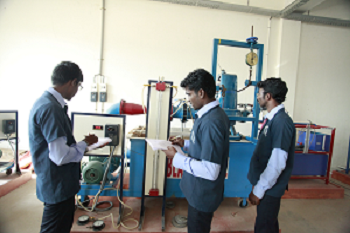Department Facilities

Fluid Mechanics and Machinery Lab

The fluid Mechanics Lab mainly focussed on determination of flow parameters related to fluid flow in pipes and open channels.The lab hleps to getting idea about calibration of venturimeter, orifice meter, notches, etc.

Upon Completion of this subject, the students can able to have hands on experience in flow measurements using different devices and also perform calculation related to losses in pipes and also perform characteristic study of pumps, turbines etc.,

List Of Experiments

1.Determination of the Coefficient of discharge of given Orifice meter.

2.Determination of the Coefficient of discharge of given Venturimeter.

3.Calculation of the rate of flow using Rota meter.

4.Determination of friction factor for a given set of pipes.

5.Conducting experiments and drawing the characteristic curves of centrifugal pump/ submergible pump

6.Conducting experiments and drawing the characteristic curves of reciprocating pump.

7.Conducting experiments and drawing the characteristic curves of Gear pump.

8.Conducting experiments and drawing the characteristic curves of Pelton wheel.

9.Conducting experiments and drawing the characteristics curves of Francis turbine.

10. Determination of the Coefficient of discharge of rectangular and triangular notches.

11. Determination of the metacentric height of given floating body.